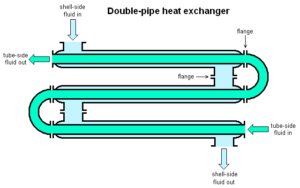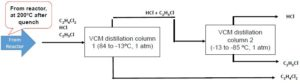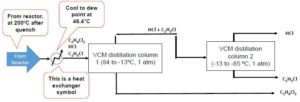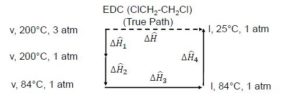# 35 Phase Change and Heat Capacity

Learning Objectives

By the end of this section, you should be able to:

Evaluate the cost of utilities in processes

Characterize energy changes in a system due to changes in temperature

Analyze energy balances on processes involving phase changes

## Energy Balance Equipment

• Almost all energy exchange equipment is either a heat exchanger or jacket
• Heat exchangers and jackets often work by transferring heat with a stream of fluid
• Electrical heating elements are also available, but their high cost per unit energy may limit their use in industry

The figure below is a shell-and-tube heat exchange:Image from Turbojet /CC BY-SA 4.0

Utilities: Utilities are services at a site such as water, electricity, and gas.

Choosing the correct utility can make a big difference in the cost of heating or cooling. For example, evaporating toluene at $190^{\circ}C$ with a heat duty of 3,000,000 kJ/hr can be heated using the following utility options:
 Utility Inlet $T (^{\circ}C)$ Outlet $T (^{\circ}C)$ P ($psia$) Cost (dollars/GJ) Cost (dollars/hr) Cost dollars/yr @ 8,000 hrs High-pressure steam 250 249 575 5.66 16.98 135,840 Electricity N/A N/A N/A 18.72 56.16 449,280

The high-pressure steam is a much cheaper option here

## Phase Changes

Phase changes take place when a compound or mixture of compounds undergoes a change in their state of matter. The enthalpy changes for phase changes occur at a constant temperature and pressure and are determined experimentally.

Parameter Phase Transition
Liquid → Gas Heat of Vapourization ($\Delta\hat{H}_{vap}$)
Solid → Liquid Heat of Fusion ($\Delta\hat{H}_{fus}$)
Gas → Liquid (-) Heat of Vapourization ($-\Delta\hat{H}_{vap}$)
Liquid → Solid (-) Heat of Fusion ($-\Delta\hat{H}_{fus}$)

Note that heat of vapourization is also referred to as latent heat

## Heat Capacities

Heat capacities are physical properties that describe how much heat is needed to increase the temperature of a compound for a unit temperature per unit mass of a compound.

For a closed system where the only changing system variable is temperature:

$Q = \Delta\hat{U}$

The heat capacity at constant volume can be described as follows, where $C_{V}$ is a function of temperature $T$:

$C_{V}(T) = \bigg(\frac{\delta\hat{U}}{\delta T}\bigg)_{V}$

Notation: $\big(\;\;\big)_{V}$ means that the volume is kept constant during the process

This expression can be rearranged and integrated to obtain the following:

 $d\hat{U} = C_{V}(T)dT$ $\Delta\hat{U} = \int^{T_{2}}_{T_{1}}C_{V}dT$

If the $C_{V}$ is constant:

$\Delta\hat{U} = C_{V}*(T_{2}-T_{1})$

For an open system, the heat capacity is defined under constant pressure conditions:

 $C_{P}(T) = \bigg(\frac{\delta\hat{H}}{\delta T}\bigg)_{P}$ $\Delta\hat{H} = \int^{T_{2}}_{T_{1}}C_{P}dT$

If the $C_{P}$ is constant:

$\Delta\hat{H} = C_{P}*(T_{2}-T_{1})$

Example: Phase Changes and Heat Capacity in Energy Balances

Consider a system where a mixture of dichloroethane ($EDC$), hydrogen chloride ($H\!Cl$), and vinyl chloride ($V\!C$) is entering a distillation column for separation. Distillation columns work based on vapor-liquid equilibrium, and therefore the column will generally operate at a temperature between the boiling points of all compounds.
 Compound Formula Boiling Point at 1 atm ($^{\circ}C$) Heat of Vapourization at 1 atm ($kJ/mol$) EDC $C_{2}H_{4}Cl_{2}$ 84 35 HCl $HCl$ -85 16 VC $C_{2}H_{3}Cl$ -13 21

The mixture exits a reactor at $200^{\circ}C$ and has the following properties:

 Compound Formula Flow to Separate (tonne/hr) Flow to Separate (tonne-mol/hr) $y_{i}$ (gas mole fraction) EDC $C_{2}H_{4}Cl_{2}$ 47.5008 0.48 0.25 HCl $HCl$ 26.2512 0.72 0.375 VC $C_{2}H_{3}Cl$ 45 0.72 0.375Recall Raoult’s Law:

$x_{i} = \frac{p_{i}*}{y_{i}×P }$

 Compound Formula Flow to Separate (tonne/hr) Flow to Separate (tonne-mol/hr) $y_{i}$ (gas mole fraction) $x_{i}$ (liquid mole fraction) EDC $C_{2}H_{4}Cl_{2}$ 47.5008 0.48 0.25 0.94 HCl $HCl$ 26.2512 0.72 0.375 0.005 VC $C_{2}H_{3}Cl$ 45 0.72 0.375 0.053

Suppose we operate the distillation column at the dew point of the system to separate benzene. For this system, the dew-point temperature is $46.4^{\circ}C$, therefore we must use a heat exchanger to bring the mixture from $200^{\circ}C$ to $46.4^{\circ}C$How much energy is removed to cool this stream from from $200^{\circ}C$ to $46.4^{\circ}C$?

The ideal gas heat capacities for the mixture described above are listed below:
 Compound Formula Flow to Separate (tonne/hr) Flow to Separate (tonne-mol/hr) Cp (J/mol-K) EDC $C_{2}H_{4}Cl_{2}$ 47.5008 0.48 29 HCl $HCl$ 26.2512 0.72 29 VC $C_{2}H_{3}Cl$ 45 0.72 29
• EDC:

$\Delta\dot{H} = \dot{n}\int^{T_{2}}_{T_{1}} C_{P}(T)dT$

$\Delta\dot{H} = 0.48\frac{tonne-mol}{h}*1,000,000\frac{mol}{tonne-mol}*29\frac{J}{mol-K}*(200^{\circ}C-46.4^{\circ})*\frac{1K}{1^{\circ}}$

$\Delta\dot{H} = 2.14 x 10^{9} \frac{J}{h}$

• HCl and VC:

$\Delta\dot{H} = \dot{n}\int^{T_{2}}_{T_{1}} C_{P}(T)dT$

$\Delta\dot{H} = 0.72\frac{tonne-mol}{h}*1,000,000\frac{mol}{tonne-mol}*29\frac{J}{mol-K}*(200^{\circ}C-46.4^{\circ})*\frac{1K}{1^{\circ}}$

$\Delta\dot{H} = 3.21 x 10^{9} \frac{J}{h}$

There is no exchange of energy in the form of work in a heat exchanger. Therefore, the total heat removed $\dot{Q}=\Sigma\Delta\dot{H}$ is the sum of the change in enthalpy for each species:

$\dot{Q}=\Sigma\Delta\dot{H}=8.56 x 10^{9} \frac{J}{h}$

Exercise: Energy Balance for a Heat Exchanger

Consider an equimolar binary mixture of n-hexane and n-heptane at a constant pressure of 1 atm flowing at 1 kmol/h. This mixture is originally at $150 ^{\circ}C$ and needs to be cooled to $85^{\circ}C$ for a process in order to ensure vapor-liquid equilibrium is satisfied. The process uses a heat exchanger to achieve this cooling. The heat capacities for both compounds can be described by the following expression:

$C_{P} = A + BT + CT^{2} + DT^{3}$

where $C_{P}$ is in J/mol-K and the constants A, B, C, and D are listed below:

 Compound A B C D n-Hexane -4.413 0.528 -3.119E-04 6.494E-8 n-Heptane -5.146 0.6762 -3.651E-04 7.658E-08

How much heat needs to be removed by the heat exchanger to reach the required temperature for the process?

### Solution

Step 1: Calculate the change in enthalpy for each compound using the heat capacities taking 1 kmol/hr as the molar flow.

$\Delta\dot{H} = \dot{n}\int^{T_{2}}_{T_{1}} C_{P}(T)dT$

where $T_{1} = 150 ^{\circ}C=423K$ and $T_{2} = 85 ^{\circ}C=358 K$

$\Delta\dot{H} = \dot{n}\int^{T_{2}}_{T_{1}} (A + BT + CT^{2} + DT^{3})dT$

$\Delta\dot{H} = \dot{n}*(AT + \frac{B}{2}T^{2} + \frac{C}{3}T^{3} + \frac{D}{4}T^{4}$

For n-hexane:

$\Delta\dot{H} = 0.5\frac{kmol}{h}*(-4.413*T + \frac{0.528}{2}T^{2} + \frac{-3.119E-04}{3}T^{3} + \frac{6.494E-8}{4}T^{4})\bigg|^{423 K}_{358 K}$

$\Delta\dot{H} = 0.5\frac{kmol}{h}*1000 \frac{mol}{kmol}*1859\frac{J}{mol}*\frac{kJ}{1000 J}$

$\Delta\dot{H} = 929.5 \frac{kJ}{h}$

Similarly for n-heptane:

$\Delta\dot{H} = 0.5\frac{kmol}{h}*1000 \frac{mol}{kmol}*2424\frac{J}{mol}*\frac{kJ}{1000 J}$

$\Delta\dot{H} = 1212 \frac{kJ}{h}$

Step 2: Sum up the enthalpy changes for the components.

\begin{align*}
\dot{Q} &= \Sigma\Delta\dot{H}\\
\dot{Q} & = (929.5+1212)\frac{kJ}{h}\\
\dot{Q} &= 2142\frac{kJ}{h}
\end{align*}

### Choosing Utilities for System

For the VCM distillation system described above, we have the following utility options for cooling the stream from $200^{\circ}C$ to $46.4^{\circ}C$:
 Option Utility Inlet T ($^{\circ}C$) Outlet T ($^{\circ}C$) P Cost (dollars/GJ) A Cooling Water 20 25 N/A 0.378 B Refrigerated Water 5 15 N/A 4.77 C Low T Refrigerant -20 -5 N/A 8.49
A rule of thumb is that we want a stream we are transferring energy to have a $10^{\circ}C$ temperature difference with the process stream. This will ensure our heat transfer occurs at an effective rate and that our heat exchanger does not need to be too big.
Since our outlet temperature is only $46.4^{\circ}C$, all options have an inlet temperature at least $10^{\circ}C$ below this desired outlet temperature. We could pick any but will select the cheapest option, which is cooling water.

How much will cooling this stream cost?

\begin{align*}
Cost \Big(\frac{dollars}{h}\Big) &= \dot{Q}*cost\Big(\frac{dollars}{GJ}\Big)\\
& = 8.56\frac{GJ}{h}*0.378\frac{dollars}{GJ}\\
& = 3.24\frac{dollars}{h}
\end{align*}

## Process Paths

Recall that $\hat{U}$ and $\hat{H}$ are state properties. These properties depend on the state and not the path to that state.

It is easier to calculate the enthalpy change by changing one variable at a time through a hypothetical process path.

1 – Calculate change in enthalpy by only changing the temperature at the same state

2 – Calculate change in enthalpy by only changing the pressure at the same state (in the course, we usually neglect the effect of pressure on enthalpy change)

3 – Calculate change in enthalpy by only changing the phase

To obtain the desired change in enthalpy, add each enthalpy change where only 1 state property changes:

 $\Delta\hat{H} = \Sigma_{i}\Delta\hat{H}_{i}$

Example: EDC Process Path

Consider the enthalpy change of $EDC$ transforming from vapour at $200^{\circ}C$ and 3 atm to liquid at $25^{\circ}C$ and 1 atm:In this process, there are 3 variables changing:

• pressure
• temperature
• phase (vapour to liquid)

The following steps are taken in the process path and added together to get the overall change:

$\Delta\hat{H}_{1}$ is the enthalpy change from going from 3 atm to 1 atm at a constant temperature of 200°C in the vapour phase ($v$)

$\Delta\hat{H}_{2}$ is the enthalpy change from going from 200°C to 84°C at a constant pressure of 1 atm in the vapour phase ($v$)

$\Delta\hat{H}_{3}$ is the enthalpy change for a phase change, going from the vapour phase ($v$) to the liquid phase ($l$) at a constant pressure and temperature of 1 atm and 84°C

$\Delta\hat{H}_{4}$ is the enthalpy change from going from 84°C to 25°C at a constant pressure of 1 atm in the liquid phase ($l$)

When we add these changes together, we can get the overall change in enthalpy:

$\Delta\hat{H} = \Delta\hat{H}_{1} + \Delta\hat{H}_{2} + \Delta\hat{H}_{3} + \Delta\hat{H}_{4}$

Exercise: Process Path

What process paths can be taken to calculate the change in enthalpy for acetone going from $25^{\circ}C$ in the liquid phase to $60^{\circ}C$ in the vapour phase?

### Solution

Step 1: Bring the acetone to the boiling point temperature from $25^{\circ}C$ without changing the phase using the $C_{P}$ in the liquid phase.

Step 2: Use the latent heat (or heat of vaporization) to calculate the enthalpy of changing the phase from liquid to vapour.

Step 3: Bring the acetone to $60^{\circ}C$ from the boiling point temperature using the $C_{P}$ in the vapour phase.